# M/M/1 Queueing System

We have already covered queueing theory basics in a previous article. In this article we will focus on M/M/1 queueing system. As we have seen earlier, M/M/1 refers to negative exponential arrivals and service times with a single server. This is the most widely used queueing system in analysis as pretty much everything is known about it. M/M/1 is a good approximation for a large number of queueing systems.

The following topics will be discussed in detail:

## Poisson Arrivals

M/M/1 queueing systems assume a Poisson arrival process. This assumption is a very good approximation for arrival process in real systems that meet the following rules:

1. The number of customers in the system is very large.
2. Impact of a single customer on the performance of the system is very small, i.e. a single customer consumes a very small percentage of the system resources.
3. All customers are independent, i.e. their decision to use the system are independent of other users.

### Cars on a Highway

As you can see these assumptions are fairly general, so they apply to a large variety of systems. Lets consider the example of cars entering a highway. Lets see if the above rules are met.

1. Total number of cars driving on the highway is very large.
2. A single car uses a very small percentage of the highway resources.
3. Decision to enter the highway is independently made by each car driver.

The above observations mean that assuming a Poisson arrival process will be a good approximation of the car arrivals on the highway. If any one of the three conditions is not met, we cannot assume Poisson arrivals. For example, if a car rally is being conducted on a highway, we cannot assume that each car driver is independent of each other. In this case all cars had a common reason to enter the highway (start of the race).

### Telephony Arrivals

Lets take another example. Consider arrival of telephone calls to a telephone exchange. Putting our rules to test we find:

1. Total number of customers that are served by a telephone exchange is very large.
2. A single telephone call takes a very small fraction of the systems resources.
3. Decision to make a telephone call is independently made by each customer.

Again, if all the rules are not met, we cannot assume telephone arrivals are Poisson. If the telephone exchange is a PABX catering to a few subscribers, the total number of customers is small, thus we cannot assume that rule 1 and 2 apply. If rule 1 and 2 do apply but telephone calls are being initiated due to some disaster, calls cannot be considered independent of each other. This violates rule 3.

### Poisson Arrival Process

Now that we have established scenarios where we can assume an arrival process to be Poisson. Lets look at the probability density distribution for a Poisson process. This equation describes the probability of seeing n arrivals in a period from 0 to t.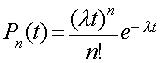Where:

• t is used to define the interval 0 to t
• n is the total number of arrivals in the interval 0 to t.
• lambda is the total average arrival rate in arrivals/sec.

### Negative Exponential Arrivals

We have seen the Poisson probability distribution. This equation gives information about how the probability is distributed over a time interval. Unfortunately it does not give an intuitive feel of this distribution. To get a good grasp of the equation we will analyze a special case of the distribution, the probability of no arrivals taking place over a given interval.

Its easy to see that by substituting n with 0, we get the following equation: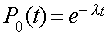This equation shows that probability that no arrival takes place during an interval from 0 to t is negative exponentially related to the length of the interval. This is better illustrated with an example.

Consider a highway with an average of 1 car arriving every 10 seconds (0.1 cars/second arrival rate). The probability distribution with t is given below. You can see here that the probability of not seeing a single car on the highway decreases dramatically with the observation period. If you observe the highway for a period of 1 second, there is 90% chance that no car will be seen during that period. If you monitor the highway for 20 seconds, there is only a 10% chance that you will not see a car on the highway. Put another way, there is only a 10% chance two cars arrive less than one second apart. There is a 90% chance that two cars arrive less than 20 seconds apart.

In the figure below, we have just plotted the impact of one arrival rate. If another graph was plotted after doubling the arrival rate (1 car every 5 seconds), the probability of not seeing a car in an interval would fall much more steeply.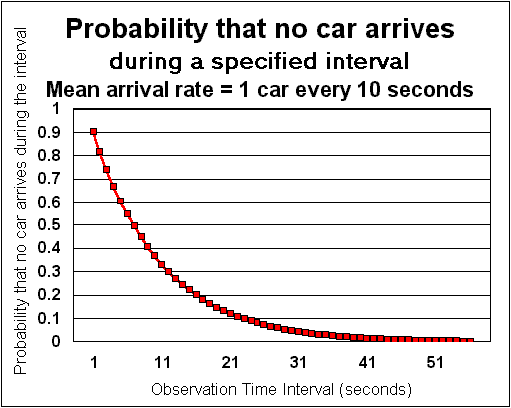## Poisson Service Times

In an M/M/1 queueing system we assume that service times for customers are also negative exponentially distributed (i.e. generated by a Poisson process). Unfortunately, this assumption is not as general as the arrival time distribution. But it could still be a reasonable assumption when no other data is available about service times. Lets see a few examples:

### Telephone Call Durations

Telephone call durations define the service time for utilization of various resources in a telephone exchange. Lets see if telephone call durations can be assumed to be negative exponentially distributed.

1. Total number of customers that are served by a telephone exchange is very large.
2. A single telephone call takes a very small fraction of the systems resources.
3. Decision on how long to talk is independently made by each customer.

From these rules it appears that negative exponential call hold times are a good fit. Intuitively, the probability of a customers making a very long call is very small. There is a high probability that a telephone call will be short. This matches with the observation that most telephony traffic consists of short duration calls. (The only problem with using the negative exponential distribution is that, it predicts a high probability of extremely short calls).

This result can be generalized in all cases where user sessions are involved.

### Transmission Delays

Lets see if we can assume negative exponential service times for messages being transmitted on a link. Since the service time on a link is directly proportional to the length of the message, the real question is that can we assume that message lengths in a protocol are negative exponentially distributed?

As a first order approximation you can assume so. But message lengths aren't really independent of each other. Most communication protocols exchange messages in a certain sequence, the length distribution is determined by the length of the messages in the sequence. Thus we cannot assume that message lengths are independent. For example, internet traffic message lengths are not distributed in a negative exponential pattern. In fact, length distribution on the internet is bi-modal (i.e. has two distinct peaks). The first peak is around the length of a TCP ack message. The second peak is around the average length of a data packet.

## Single Server

With M/M/1 we have a single server for the queue. Suitability of M/M/1 queueing is easy to identify from the server standpoint. For example, a single transmit queue feeding a single link qualifies as a single server and can be modeled as an M/M/1 queueing system. If a single transmit queue is feeding two load-sharing links to the same destination, M/M/1 is not applicable. M/M/2 should be used to model such a queue.

## M/M/1 Results

As we have seen earlier, M/M/1 can be applied to systems that meet certain criteria. But if the system you are designing can be modeled as an M/M/1 queueing system, you are in luck. The equations describing a M/M/1 queueing system are fairly straight forward and easy to use.

First we define p, the traffic intensity (sometimes called occupancy). It is defined as the average arrival rate (lambda) divided by the average service rate (mu). For a stable system the average service rate should always be higher than the average arrival rate. (Otherwise the queues would rapidly race towards infinity). Thus p should always be less than one. Also note that we are talking about average rates here, instantaneous arrival rate may exceed the service rate. Over a longer time period, the service rate should always exceed arrival rate.

ρ = λ / µ

Mean number of customers in the system (N) can be found using the following equation: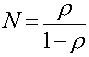You can see from the above equation that as p approaches 1 number of customers would become very large. This can be easily justified intuitively. p will approach 1 when the average arrival rate starts approaching the average service rate. In this situation, the server would always be busy hence leading to a queue build up (large N).

Lastly we obtain the total waiting time (including the service time):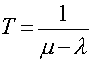Again we see that as mean arrival rate (lambda) approaches mean service rate (mu), the waiting time becomes very large.

An important lesson to learn here is that systems should be designed so that even at peak throughput of the system, resource occupancy should be a little below 100%. This is required to keep the queue lengths and delays within bounds.